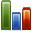# Statistics BSC304Improve your Ability to Collect, Analyze and Communicate Complex Statistical Data

Statistics is an essential tool in many areas of research including psychology and the sciences. This course provides the most essential knowledge and skills required by consultants and researchers in a wide variety of disciplines. Topics covered include measures of central tendency, correlation, regression t-test, inferential statistics such as chi square and ANOVA.

Course Structure and Content

There are ten lessons in this course as outline below:

1. Introduction

• Key terms and concepts: data, variables
• Measurements of scale: nominal, ordinal, interval,ratio
• Data presentation
• Probability
• Rounding of data
• Scientific notation
• Significant figures
• Functions
• Equations
• Inequalities
• Experimental design
• The normal curve
• Data collection
• Simple, systemic, stratified and cluster random sampling
• Remaining motivated to learn statistics

2. Distributions

• Scope and nature of distributions
• Class intervals and limits
• Class boundaries
• Frequency Distribution
• Histograms
• Frequency polygons
• Normal distributions
• Other distributions
• Frequency curves

3. Measures of central tendency

• Range, percentiles, quartiles, mode, median, mean
• Variance
• Standard deviation
• Degrees of freedom
• Interquartile and semi interquartile deviations

4. The Normal curve and Percentiles and Standard Scores

• Normal distribution characteristics
• Percentiles
• Standard scores
• Z scores
• T score
• Converting standard scores to percentiles
• Area under a curve
• Tables of normal distribution

5. Correlation

• Scope and nature of Correlation
• Correlation coefficient
• Coefficient of determination
• Scatter plots
• Product movement for linear correlation coefficient
• Rank correlation
• Multiple correlation

6. Regression

• Calculating regression equation with correlation coefficient
• Least squares method
• Standard error of the estimate

7. Inferential Statistics

• Hypothesis testing
• Test for a mean
• Errors in accepting or rejecting null hypothesis
• Levels of significance
• One and two tailed tests
• Sampling theory
• Confidence intervals

8. The t Test

• Assessing statistical difference with the t test
• t Test for independent samples
• t Test for dependent (paired) samples

9. Analysis of variance

• Scope and application of ANOVA
• Factors and levels
• Hypothesis
• Calculate degrees of freedom
• Calculate sum of squares within and between groups
• Calculate mean square
• Calculate F

10. Chi square test

• Chi square goodness of fit test
• Calculate degrees of freedom
• Chi square test of independence
• Calculate expected frequencies
• Degrees of freedom
• Contingency tables
• Find expected frequencies
• Calculate degrees of freedom

### Using Statistics

Collecting statistical information is the starting point for any task involving statistics; but alone, there is little point to collecting the information. It is only when statistics are organized, analyzed and communicated to others, that they really find value.

A good statistician needs to be a communicator; and there are many different tools which can be used to communicate statistical data to others. One of the most common tools is to use a graph.

Graphs are used to show proportional relationships and trends. The most commonly used types of graph are:

• Pie chart - a circle divided into sections, with each section representing a percentage of the whole
• Bar graph - vertical or horizontal bars that show a comparison between categories.
• Line graph - a line that links points plotted in relation to two axes drawn at right angles. A line graph shows trends or the change of one or more variables over time.
• Flow chart - a chart that displays the major steps and links in a process.

Some tips for preparing graphs:

• Place a simple and descriptive title directly above or below the graph.
• Use an appropriate scale, e.g. if an age group is 0 to 10 years, don’t show a scale of 0 to 20 years.
• Keep the design simple.
• Prepare a separate graph for each point.
• Tie the graph to the text, refer to it in the text, and place it as close to the text as possible.
• Limit the graphs to two or three sizes within a document, using the table outline (in two or three sizes) to create a sense of uniformity.

### Enrol Now!

Fee Information (S3)
Prices in Australian Dollars

 Plan Aust. Price Overseas Price A 1 x \$878.90 1 x \$799.00 B 2 x \$475.20 2 x \$432.00

Note: Australian prices include GST.